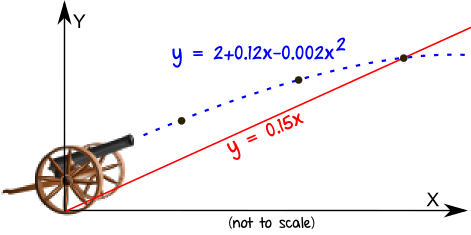By the end of this topic you will be able to:
• Given the nature of the roots of a quadratic equation, use the discriminant to find an unknown
• Finding the coordinates of the point(s) of intersection of a straight line and a curve or of two curves
• know condition for tangency; intersection of line and parabola (lines and curves)

Due: Wednesday 2nd September

Please check the solutions below and attempt the equivalent questions from the Set 2 sheet to those you struggled with first time.  These should be handed in with your next homework or earlier. Please see me for help if necessary.

🙂New Topic: Quadratic Theory, MiA Unit 2 Ch1.2

By the end of this topic you will be able to:
• use the quadratic formula to calculate roots
• know that discriminant of ax² + bx + c = 0 is b² – 4ac
• use discriminant to determine nature of roots of a quadratic
• use discriminant to find condition that the roots of a quadratic are real, equal or unequal
• know condition for tangency; intersection of line and parabola (lines and curves)
• determine a quadratic equation given roots